# 使用 SUMO 进行仿真(1)-快速入门2021年9月23日07:45:32

5 4679字阅读15分35秒

## 简介

1. pip install -U -i https://test.pypi.org/simple/ eclipse-sumo
2. pip install -U -i https://test.pypi.org/simple/ traci sumolib libsumo

## Hello SUMO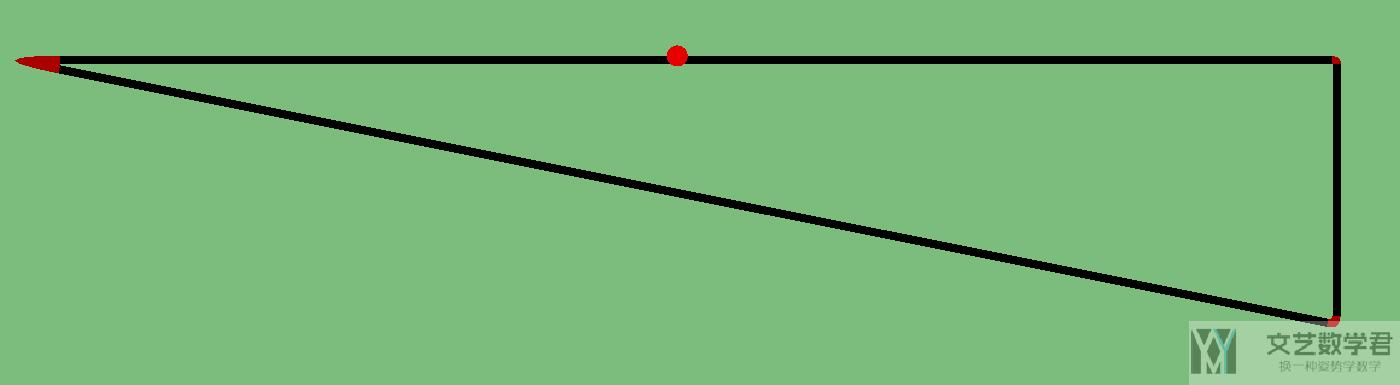### Node 文件介绍

1. <nodes>
2.     <node id="1" x="-250.0" y="0.0" />
3.     <node id="2" x="+0.0" y="0.0" />
4.     <node id="3" x="+250.0" y="0.0" type="traffic_light" />
5.     <node id="4" x="+250.0" y="-100.0" />
6. </nodes>

2.     <tlLogic id="3" type="static" programID="0" offset="0">
3.         <phase duration="3" state="G"/>
4.         <phase duration="1"  state="y"/>
5.         <phase duration="3" state="r"/>
6.     </tlLogic>

`.additional.xml`文件除了可以设置信号灯时长之外，还可以设置探测器等。这些我们会在后面进行介绍。

### Edge 文件介绍

1. <edges>
2.     <edge from="1" id="1to2" to="2" />
3.     <edge from="2" id="2to3" to="3" />
4.     <edge from="3" id="3to4" to="4" />
5.     <edge from="1" id="1to4" to="4" />
6. </edges>

### Network 的介绍

1. netconvert --node-files=hello.nod.xml --edge-files=hello.edg.xml --output-file=hello.net.xml

1. netconvert --node-files=hello.nod.xml --edge-files=hello.edg.xml --tllogic-files=hello.additional.xml --output-file=hello.net.xml#### 查看信号灯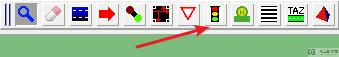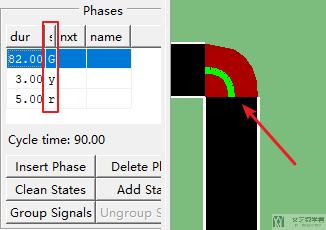#### 查看车道 ID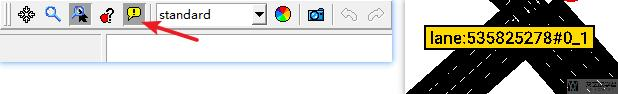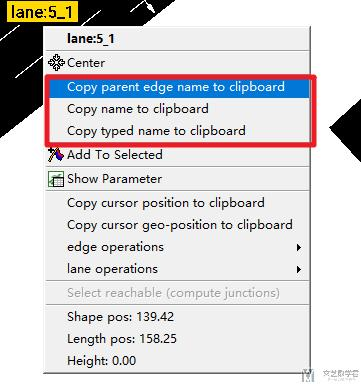### Route 文件的介绍

1. <routes>
2.     <vType accel="1.0" decel="1.0" id="Car1" length="5.0" maxSpeed="10.0" sigma="0.0" /> <!--id表示第一种类型的车-->
3.     <vType accel="5.0" decel="1.0" id="Car2" length="7.0" maxSpeed="10.0" sigma="0.0" /> <!--id表示第一种类型的车-->
4.     <route id="route0" edges="1to2 2to3 3to4"/>
5.     <route id="route1" edges="1to4"/>
6.     <vehicle depart="1" id="veh0" route="route0" type="Car1" />
7.     <vehicle depart="2" id="veh1" route="route1" type="Car2" />
8. </routes>

### Configuration

1. <viewsettings>
2.     <viewport y="0" x="250" zoom="100"/>
3.     <delay value="100"/>
4. </viewsettings>

1. <configuration>
2.     <input>
3.         <net-file value="hello.net.xml"/>
4.         <route-files value="hello.rou.xml"/>
5.         <gui-settings-file value="hello.settings.xml"/>
6.     </input>
7.     <time>
8.         <begin value="0"/>
9.         <end value="10000"/>
10.     </time>
11. </configuration>

1. sumo-gui -c hello.sumocfg

• `time-to-teleport`，车辆转移（消失）的时间。如果车辆停止超过一定时间，就会自动消失；
• `no-warnings`，不显示 warning；
• `no-step-log`，不在终端显示仿真过程的信息，仿真步数等；
1. sumo -c hello.sumocfg -r 10000.rou.xml --time-to-teleport 1000 --no-warnings --no-step-log

`GUI` 的界面内，我们可以选择模式。这里我们选择是 `real world`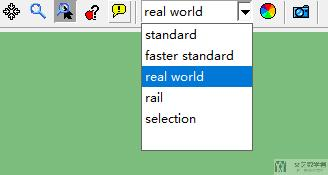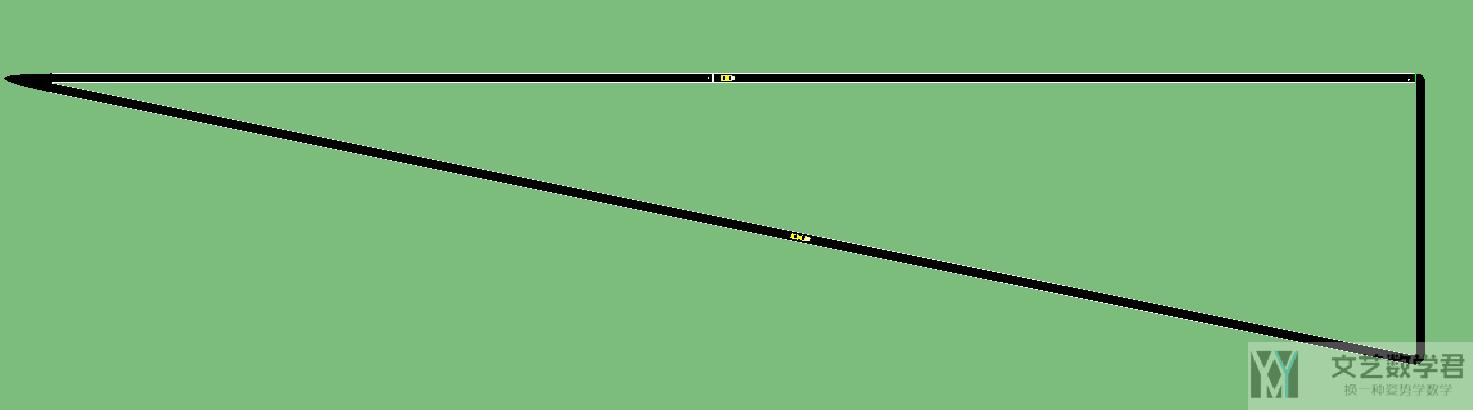## NetEdit 的使用介绍

### 查看信号灯的信息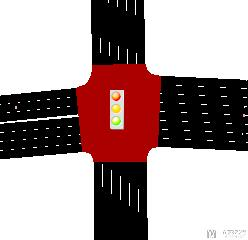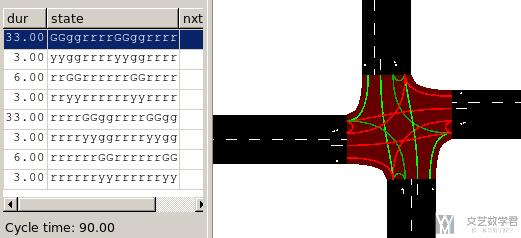### 修改车道之间的 connection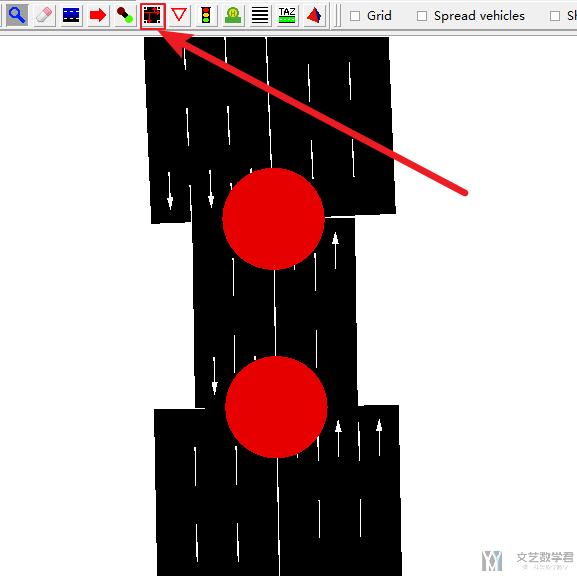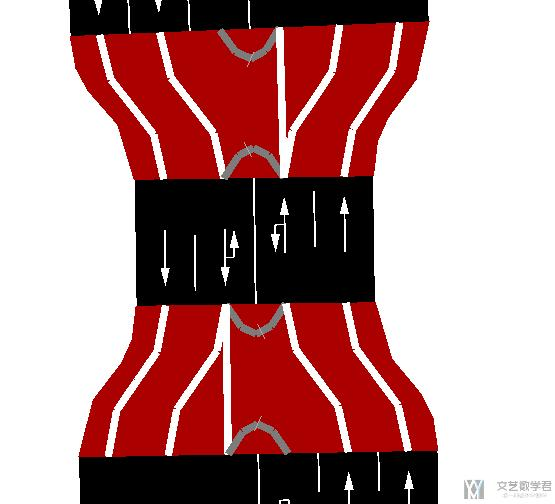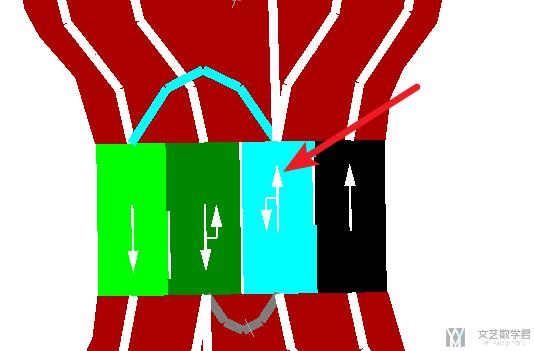## 一些存在的问题

### 在 SUMO GUI 中无法输入文字

• 微信公众号
• 关注微信公众号
•• QQ群
• 我们的QQ群号
•• 本文由 发表于 2021年9月23日07:45:32
• 转载请务必保留本文链接：https://mathpretty.com/12816.html